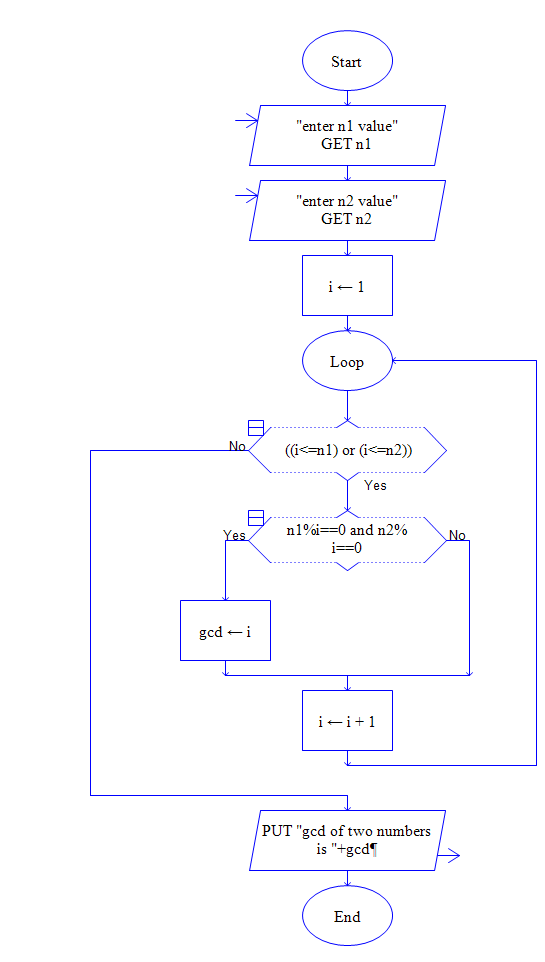The Flowchart given here represents the calculation of GCD (Greatest Common Divisor).The above flowchart is drawn in the Raptor tool. The flowchart represents the flow for finding Greatest Common Divisor

Example: GCD of two numbers 12 & 24 is 12

The code for calculating the LCM and GCD is given in the below link.

Finding GCD and LCM of Given Numbers using C